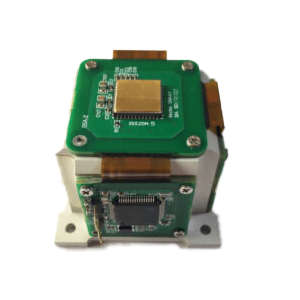Application

# IMU inertial measurement unit (provides odometer, position, attitude)1.Introduction to Inertial Technology

(1) Inertial device – mechanical gyroscope

Fixed axis: When the gyro rotor rotates at a high speed, when there is no external moment acting on the gyro, the orientation of the rotation axis of the gyro in the inertial space remains stable, that is, it points to a fixed direction; at the same time, it resists any The force that changes the axial direction of the rotor.

Precession: When the rotor rotates at high speed, if the external torque acts on the outer ring axis, the gyroscope will rotate around the inner ring axis; if the external torque acts on the inner ring axis, the gyroscope will rotate around the outer ring axis. The direction of the rotational angular velocity and the direction of the external torque are perpendicular to each other.

(2) Inertial device – laser gyro

The Sagnac effect was discovered by the French physicist Sagnac in 1913. Its principle is that when the interferometer is stationary relative to the inertial space, the optical paths of the optical paths A and B are equal. When there is an angular velocity, the optical paths are not equal, and interference will occur.

(3) Inertial device – fiber optic gyroscope

Also based on the Sagnac effect, the propagation medium is changed to optical fiber.

(4) Inertial device – MEMS gyroscope

Coriolis force (referred to as Coriolis force) is the deviation of the linear motion of the mass point in a rotating system (such as the autobiographical earth, rotating disk, etc.) from the linear motion generated by the inertia relative to the rotating system. a description of the shift.

(5) Inertial device – accelerometer

When the carrier performs acceleration movement relative to the inertial space, the instrument housing also performs relative movement, and the mass maintains the inertia and produces displacement (stretching or compressing the spring) in the opposite direction to the acceleration direction. When the displacement reaches a certain value, the force given by the spring causes the mass block to accelerate relative to the inertial space with the same acceleration, and the magnitude and direction of the acceleration affect the direction and stretch of the mass block relative displacement.

2.Inertial device error analysis

(1) Quantization noise

The inherent noise of all quantization operations is the inevitable noise of digital sensors;

Cause: In the process of collecting continuous time signals into discrete signals through AD acquisition, the accuracy will be lost. The size of the accuracy loss is related to the step size of AD conversion. The smaller the step size, the smaller the quantization noise.

(2) Angle random walk

Broadband angular rate white noise: The output angular rate of the gyro contains noise. The white noise component in the noise and the error of the Markov property contained in the angle error are called angle random walk.

Reason: The essence of calculating the attitude is to integrate the angular rate, which must also integrate the noise. The integral of white noise is not white noise, but a Markov process, that is, the error at the current moment is obtained by accumulating a random white noise on the basis of the error at the previous moment.

(3) Angular rate random walk

Similar to the angular random walk, the error of the Markov property contained in the angular rate error is called the angular rate random walk. And this Markov error is the result of accumulation of broadband angular acceleration white noise.

(4) Zero bias instability noise

Zero bias: the so-called bias, which is generally not a fixed parameter, but drifts slowly and randomly within a certain range.

Zero bias instability: The zero bias changes slowly with time, and its change value cannot be predicted. It is necessary to assume a probability interval to describe how likely it is to fall within this interval. The longer the time, the larger the interval.

(5) Rate ramp

This error is a trend error, not a random error. Random error means that you cannot use a deterministic model to fit and eliminate it. At most, you can only use a probabilistic model to describe it. The prediction results obtained in this way are also probabilistic, and the trend error can be directly eliminated by fitting. The most common cause of this error in a gyroscope is the zero position change caused by temperature, which can be eliminated by temperature compensation.

(6) Zero offset repeatability

Over multiple starts, the zero offsets are not equal, so there is a repeatability error. In actual use, it needs to be re-estimated every time the power is turned on. Allan variance analysis does not include the analysis of zero bias repeatability.

3.Internal parameter calibration of inertial devices

Due to processing reasons, zero offset, scale factor error, and installation error occur.

Calibration method

(1)Internal parameter error calibration of inertial device

(2)Discrete level calibration

(3)Semi-system level calibration

(4)System level calibration

Comparison of Different Types of Calibration Methods

(1)The discrete-level calibration accuracy is higher, but it depends on the turntable.

(2)The calibration accuracy at the semi-system level is the worst, but it does not depend on the turntable, with low cost and high efficiency, and the calibration requirements for MEMS are sufficient.

(3)The system-level calibration has the highest accuracy, but it is only suitable for calibrating high-precision inertial navigation.

4.Inertial device temperature compensation

The essence of temperature compensation is system identification, which not only needs to find a suitable physical model, but also identifies the parameters of the physical model.

Temperature compensation is the most important in device error compensation, but it is also the most “non-technical”

Inertial navigation calculation includes: attitude calculation, speed calculation, and position calculation, among which attitude calculation is the core.